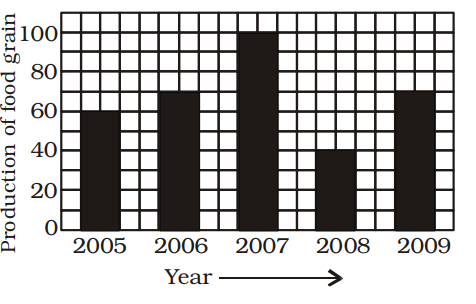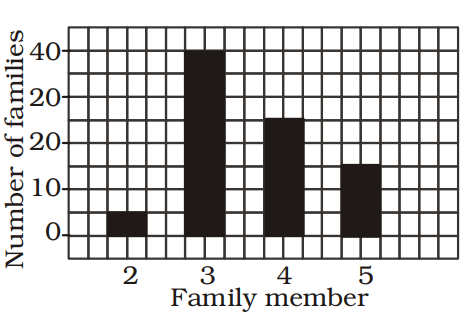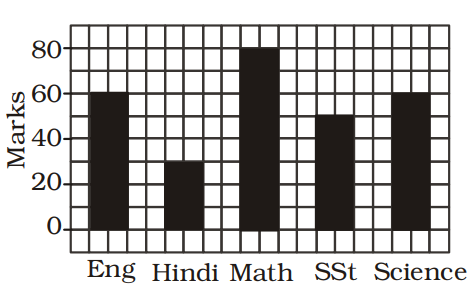## Introduction to Data Interpretation

#### Data Interpretation

Direction: Read the following pie-chart to answer the questions given below it.1. If the total amount spent was \$ 46000/-, how much was spent on clothing and housing together?

1. Total amount spent = 46,000
Amount spent clothing and housing together = (10% + 15%) of
\$ 46000

 = 25 × 46,000 = \$ 11500 100

##### Correct Option: A

Total amount spent = 46,000
Amount spent clothing and housing together = (10% + 15%) of
\$ 46000

 = 25 × 46,000 = \$ 11500 100

1. If the total amount spent during the year 1998 was \$ 46000/-, the amount spent on food, was :

1. Total amount spent = \$ 46,000
Amount spent on food = 23% of 46,000

 = 23 × 46,000 = \$ 10580 100

##### Correct Option: B

Total amount spent = \$ 46,000
Amount spent on food = 23% of 46,000

 = 23 × 46,000 = \$ 10580 100

1. Study the above bar graph showing the production of food grains (in million tons). What is the ratio between the maximum production and the minimum production during the given period?1. According to given bar graph , we see
The maximum production of food grains in 2007 = 100 million tonnes
The minimum production of food grains in 2008 = 40 million tonnes
∴ Required ratio = The maximum production of food grains in 2007 : The minimum production of food grains in 2008

##### Correct Option: D

According to given bar graph , we see
The maximum production of food grains in 2007 = 100 million tonnes
The minimum production of food grains in 2008 = 40 million tonnes
∴ Required ratio = The maximum production of food grains in 2007 : The minimum production of food grains in 2008
Required ratio = 100 : 40 = 5 : 2

1. Study the bar graph carefully and answer the following question. Which type of family is the most common?1. As per the given above bar graph , we see that
3 members type of family is the most common , which have number of families 40.

##### Correct Option: B

As per the given above bar graph , we see that
3 members type of family is the most common , which have number of families 40.
Hence , required answer is option B .

1. The above bar graph show the marks obtained by a student in an examination. What is the average marks obtained by the student?1. According to given bar graph , we have
Total marks obtained by a student in all 5 subjects = 60 + 30 + 80 + 50 + 60 = 280
Number of given subjects = 5

 Required Average marks = Total marks obtained by a student in all 5 subjects Number of given subjects

##### Correct Option: B

According to given bar graph , we have
Total marks obtained by a student in all 5 subjects = 60 + 30 + 80 + 50 + 60 = 280
Number of given subjects = 5

 Required Average marks = Total marks obtained by a student in all 5 subjects Number of given subjects

 Required Average marks = 280 = 56 5